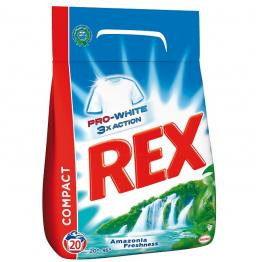# One-fifth 4469

Two hundred boxes of washing powder were lined up in 3 rows in the store. There were 13 more boxes in the first row than in the second row, one-fifth more in the second row than in the third row. How many boxes were there in each row?

a =  79
b =  66
c =  55

### Step-by-step explanation:

a+b+c=200
a = 13 + b
b = c+ 1/5·c

a+b+c = 200
a-b = 13
5b-6c = 0

Row 2 - Row 1 → Row 2
a+b+c = 200
-2b-c = -187
5b-6c = 0

Pivot: Row 2 ↔ Row 3
a+b+c = 200
5b-6c = 0
-2b-c = -187

Row 3 - -2/5 · Row 2 → Row 3
a+b+c = 200
5b-6c = 0
-3.4c = -187

c = -187/-3.4 = 55
b = 0+6c/5 = 0+6 · 55/5 = 66
a = 200-b-c = 200-66-55 = 79

a = 79
b = 66
c = 55

Our linear equations calculator calculates it.Did you find an error or inaccuracy? Feel free to write us. Thank you!

Tips for related online calculators
Do you have a system of equations and looking for calculator system of linear equations?